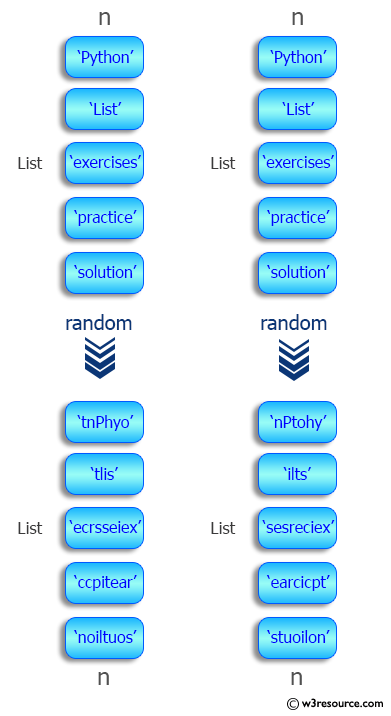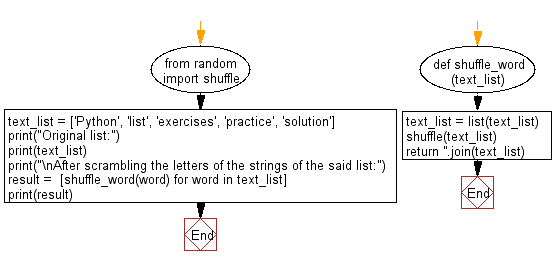﻿ Python: Scramble the letters of string in a given list - w3resource# Python: Scramble the letters of string in a given list

## Python List: Exercise - 98 with Solution

Write a Python program to scramble the letters of string in a given list.

Sample Solution:

Python Code:

``````from random import shuffle

def shuffle_word(text_list):
text_list = list(text_list)
shuffle(text_list)
return ''.join(text_list)

text_list = ['Python', 'list', 'exercises', 'practice', 'solution']
print("Original list:")
print(text_list)
print("\nAfter scrambling the letters of the strings of the said list:")
result =  [shuffle_word(word) for word in text_list]
print(result)
```
```

Sample Output:

```Original list:
['Python', 'list', 'exercises', 'practice', 'solution']

After scrambling the letters of the strings of the said list:
```

Pictorial Presentation:Flowchart:## Visualize Python code execution:

The following tool visualize what the computer is doing step-by-step as it executes the said program:

Python Code Editor:

Have another way to solve this solution? Contribute your code (and comments) through Disqus.

What is the difficulty level of this exercise?

Test your Python skills with w3resource's quiz

﻿

## Python: Tips of the Day

Floor Division:

When we speak of division we normally mean (/) float division operator, this will give a precise result in float format with decimals.

For a rounded integer result there is (//) floor division operator in Python. Floor division will only give integer results that are round numbers.

```print(1000 // 300)
print(1000 / 300)```

Output:

```3
3.3333333333333335```